# Physical Chemistry : Atoms and Elements

## Example Questions

← Previous 1 3 4

### Example Question #1 : Atomic Nucleus

How many neutrons are present in the nucleus of one atom of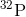Explanation:

From the periodic table, we find that the atomic number, i.e., the number of protons in the nucleus of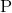is 15. Given that the mass number is 32, and recalling the formula which relates mass number to the number of neutrons and protons: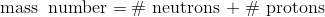, we find that the number of neutrons in the nucleus of one atom ofis 17.

### Example Question #1 : Atoms And Elements

Which atomic symbol represents a period five transition metal that has 42 electrons when it forms a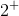cation?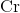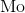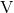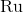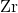Explanation:

Transition metals extend from groups 3 through 12 and periods 4 through 7. Ru, in its neutral state, has 44 electrons. Therefore, when it becomes acation, it will have 42 electrons.

### Example Question #1 : Atomic Nucleus

What is the mass number, atomic number, and charge, of the isotope of an atom that contains 34 protons, 36 neutrons, and 36 electrons?

Mass number:Atomic number:Charge:Mass number:Atomic number:Charge: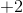Mass number:Atomic number:Charge:Mass number:Atomic number:Charge:Mass number:Atomic number:Charge:Mass number:Atomic number:Charge:Explanation:

The atomic number is equal to the number of protons in the element, so from the periodic table, we find that the element with atomic number 34 is selenium. Since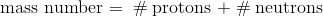, we calculate that the mass number is 70. Lastly, there are two more electrons than protons, so the charge will be.

### Example Question #1 : Atoms And Elements

Which of the following organizes the forces from strongest to weakest?

Van der Waals forces, hydrogen bonding, covalent bonding, dipole-dipole interactions

Van der Waals forces, dipole-dipole interactions, hydrogen bonding, covalent bonding

Covalent bonding, hydrogen bonding, dipole-dipole interactions, van der Waals forces

Hydrogen bonding, covalent bonding, dipole-dipole interactions, van der Waals forces

Dipole-dipole interactions, hydrogen bonding, covalent bonding, van der Waals forces

Covalent bonding, hydrogen bonding, dipole-dipole interactions, van der Waals forces

Explanation:

Covalent bonds are by far the strongest, requiring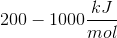to be broken.

Next are hydrogen bonds, which require between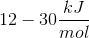to be broken.

Next are dipole-dipole interactions, which require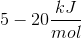to be broken.

Finally, van der Waals forces are the weakest of those listed, requiring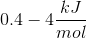to be broken.

### Example Question #2 : Atoms And Elements

Which of the following electron configurations indicates an atom in an excited state?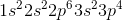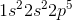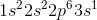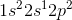Explanation:

An atom is considered to be in an excited state when one of the electrons has jumped to a higher energy level while a lower energy level is available. In the case of, an electron has jumped to the 2p energy level while there is still room in the lower 2s subshell. As a result, it is considered to be in an excited state.

### Example Question #3 : Atoms And Elements

What is the complete ground state electron configuration for the magnesium atom?

1s22s23s2

1s42p63s2

1s22s22p63s6

1s22s22p23s2

1s22s22p63s2

1s22s22p63s2

Explanation:

Magnesium has an atomic number of 12, so the total number of electrons in its configuration should add up to twelve. The maximum number of electrons in the s subshell is two. Of all the answer choices, only 1s22s22p63s2 fits the criteria. The sum of the exponent values is 12, matching the atomic number of magnesium, and the number of electrons in the s and p subshells matches the maximum amount possible.

### Example Question #1 : Electron Configuration

What is the complete ground state electron configuration for the iron atom?

1s22s22p63s23p64s23d4

1s22s22p63s23p63d6

1s22s22p63s23p64s23d6

1s42s22p63s23p24s23d6

1s22s22p63s24s23d6

1s22s22p63s23p64s23d6

Explanation:

Iron has an atomic number of 26, so the total number of electrons in its configuration should add up to twenty six. The maximum number of electrons in the s subshell is two. The sum of the exponent values is 26, matching the atomic number of magnesium, and the number of electrons in the s and p subshells matches the maximum amount possible.

### Example Question #1 : Atoms And Elements

In an atom or molecule, why can't two electrons have the same four electronic quantum numbers?

Heisenberg Uncertainty Principle

Kinetic energy operator

The first law of thermodynamics

The Pauli Exclusion Principle

Harmonic Reaction Orders

The Pauli Exclusion Principle

Explanation:

The Pauli Exclusion Principle explains various phenomena such as the structure of atoms and how different atoms combine to share electrons. When you have two electrons that are located in the same orbital, the quantum numbers n, l and ml are the same. However, ms will be different. Two electrons cannot have the same four electronic quantum numbers because no more than two electrons may occupy an orbital, and if they do, the spin of one must cancel the spin of the other so their spins will have a zero net spin angular momentum.

### Example Question #1 : Orbitals And Hybridization

What is the hybridization on the nitrogen atom in a molecule of ammonia?

sp3d

sp

sp3

sp2

sp3

Explanation:

The hybridization of an atom can be determined by the number of atoms it is bonded to, as well as the number of lone pairs it has. Two of these variables would be sp, three variables would be sp2, and four would be sp3.

The nitrogen in ammonia is bonded to three atoms of hydrogen, but also has a lone pair in order to satisfy its octet. This means that nitrogen exhibits sphybridization.

### Example Question #1 : Orbitals And Hybridization

Which of the following are true regardingandorbitals?

I. Bothandorbitals can contain a maximum of two electrons

II. In a given shell,orbitals are more numerous because they are spherical in shape

III.orbitals have lower energy thanorbitals within the same shell

I only

I and III

I and II

III only

Orbitals are regions in an electron shell where electrons might be located. There are several types of orbitals such as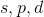, and. Most elements found on the periodic table contain electrons within one of these orbitals. A characteristic of an orbital is that it can only contain two electrons maximum. A shell might contain multiple orbitals; however, each orbital can only contain two electrons. Each orbital has a unique shape that corresponds to the electron density (the possible location of an electron at a given point in time). Theorbital has a spherical shape whereas theorbital has a dumbbell shape. As mentioned, a shell can contain multiple types of orbitals. A shell can typically contain oneorbital, threeorbitals, fiveorbitals, and sevenorbitals. Remember that the shape of the orbital has no bearing on the amount of orbitals in a shell. An orbital is higher in energy if it is found farther away from the nucleus. The orbitals in order of increasing energy is as follows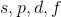. Therefore, anorbital has lower energy than aorbital in the same shell.